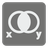Boolean Algebra
Simple
EL UK FR JA IT RU DE English ES

In mathematics and mathematical logic, Boolean algebra is a sub-area of algebra in which the values of the variables are true or false, typically denoted with 1 or 0 respectively. Instead of elementary algebra where the values of the variables are numbers and the main operations are addition and multiplication, the main operations of Boolean algebra are the conjunction (denoted ∧), the disjunction (denoted ∨) and the negation (denoted ¬).

In this mission you should implement some boolean operations:
- "conjunction" denoted x ∧ y, satisfies x ∧ y = 1 if x = y = 1 and x ∧ y = 0 otherwise.
- "disjunction" denoted x ∨ y, satisfies x ∨ y = 0 if x = y = 0 and x ∨ y = 1 otherwise.
- "implication" (material implication) denoted x→y and can be described as ¬ x ∨ y. If x is true then the value of x → y is taken to be that of y. But if x is false then the value of y can be ignored; however the operation must return some truth value and there are only two choices, so the return value is the one that entails less, namely true.
- "exclusive" (exclusive or) denoted x ⊕ y and can be described as (x ∨ y)∧ ¬ (x ∧ y). It excludes the possibility of both x and y. Defined in terms of arithmetic it is addition mod 2 where 1 + 1 = 0.
- "equivalence" denoted x ≡ y and can be described as ¬ (x ⊕ y). It's true just when x and y have the same value.

Here you can see the truth table for these operations:

x | y | x∧y | x∨y | x→y | x⊕y | x≡y |
--------------------------------------
0 | 0 |  0  |  0  |  1  |  0  |  1  |
1 | 0 |  0  |  1  |  0  |  1  |  0  |
0 | 1 |  0  |  1  |  1  |  1  |  0  |
1 | 1 |  1  |  1  |  1  |  0  |  1  |
--------------------------------------

You are given two boolean values x and y as 1 or 0 and you are given an operation name as described earlier. You should calculate the value and return it as 1 or 0.

Input: Three arguments. X and Y as 0 or 1. An operation name as a string.

Output: The result as 1 or 0.

Example:

boolean(1,...
You should be an authorized user in order to see the full description and start solving this mission.
Settings
Code:
CheckiO Extensions

CheckiO Extensions allow you to use local files to solve missions. More info in a blog post.

In order to install CheckiO client you'll need installed Python (version at least 3.8)

Install CheckiO Client first:

pip3 install checkio_client

checkio --domain=js config --key=

Sync solutions into your local folder

checkio sync

(in beta testing) Launch local server so your browser can use it and sync solution between local file end extension on the fly. (doesn't work for safari)

checkio serv -d

Alternatevly, you can install Chrome extension or FF addon

checkio install-plugin
checkio install-plugin --ff
checkio install-plugin --chromium

Read more here about other functionality that the checkio client provides. Feel free to submit an issue in case of any difficulties.

Sync...